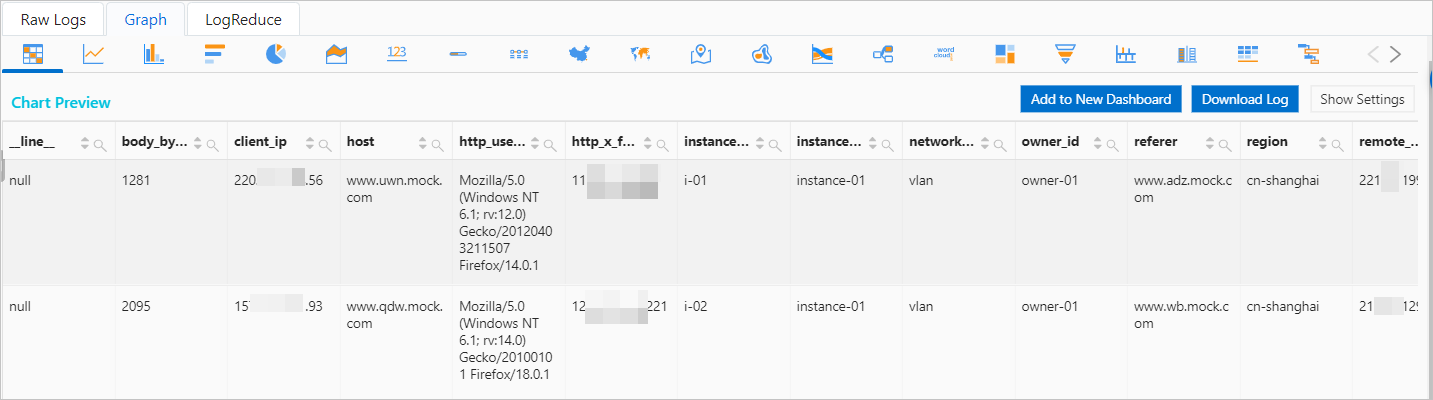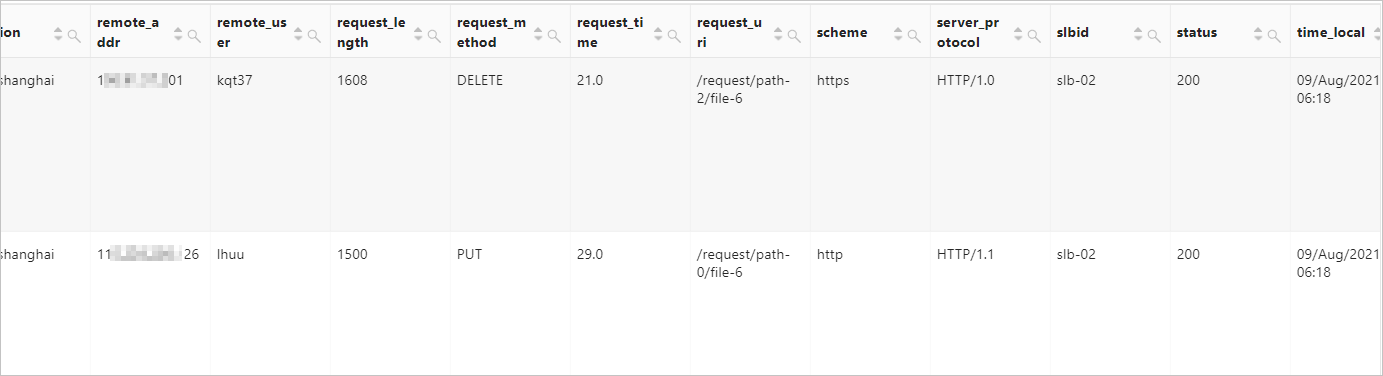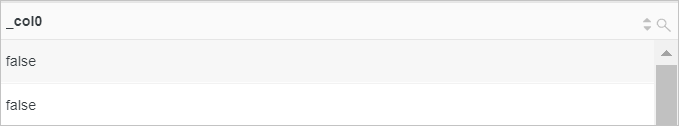Comparison operators are used to compare parameter values. The values that are of the following data types can be compared: double, bigint, varchar, timestamp, and date. This topic describes the syntax of comparison operators. This topic also provides examples on how to use the comparison operators.

Notice If you want to use strings in analytic statements, you must enclose strings in single quotation marks (''). Strings that are not enclosed or enclosed in double quotation marks ("") indicate field names or column names. For example, 'status' indicates the status string, and status or "status" indicates the status log field.
Operator Syntax Description
Relational operators x < y If x is less than y, true is returned.
x > y If x is greater than y, true is returned.
x <= y If x is less than or equal to y, true is returned.
x >= y If x is greater than or equal to y, true is returned.
x = y If x is equal to y, true is returned.
x <> y If x is not equal to y, true is returned.
x != y If x is not equal to y, true is returned.
all operator x <Relational operator> all(subquery) If x meets all conditions, true is returned.
any operator x <Relational operator> any(subquery) If x meets one of the conditions, true is returned.
between operator x between y and z If x is between y and z, true is returned.
distinct operator x is distinct from y If x is not equal to y, true is returned.
x is not distinct from y If x is equal to y, true is returned.
like operator x like pattern [escape 'escape_character'] Is used to match a specified character pattern in a string. The string is case-sensitive.
some operator x <Relational operator> some(subquery) If x meets one of the conditions, true is returned.
greatest operator greatest(x,y...) Is used to obtain the greater value of x and y.
least operator least(x,y...) Is used to obtain the smaller value of x and y.
null operator x is null If x is null, true is returned.
x is not null If x is not null, true is returned.

Relational operators

Relational operators are used to compare x and y. If the condition is met, true is returned.

Syntax

Syntax Description
x < y x is less than y.
x > y x is greater than y.
x <= y x is less than or equal to y.
x >= y x is greater than or equal to y.
x = y x is equal to y.
x <> y x is not equal to y.
x != y x is not equal to y.

Parameters

Parameter Description
x The value of this parameter is of a data type that supports comparison.
y The value of this parameter is of a data type that supports comparison.

Return value type

The Boolean type.

Examples

• Example 1: Query logs from the previous day.
• Query statement
* |
SELECT
*
FROM  log
WHERE
__time__ < to_unixtime(current_date)
AND __time__ > to_unixtime(date_add('day', -1, current_date))
• Query and analysis results• Example 2: E-commerce Company A uses the mobile and client_ip fields in access logs to find the customers whose phone numbers are from a different place than the IP addresses of the accessed websites.
• Sample field
mobile:1881111****
client_ip:192.168.2.0
• Query statement
* |
SELECT
mobile,
client_ip,
count(*) as PV
WHERE
mobile_city(mobile) != ip_to_city(client_ip)
AND ip_to_city(client_ip) != ''
GROUP BY
client_ip,
mobile
ORDER BY
PV DESC
• Query and analysis resultsall operator

The all operator is used to determine whether x meets all conditions. If all conditions are met, true is returned.

Syntax

x <Relational operator> all(subquery)

Parameters

Parameter Description
x The value of this parameter is of a data type that supports comparison.
Relational operator The value of this parameter is a relational operator. Valid values: < > <= >= = <> !=
Notice The all operator must follow the relational operator. Relational operators: < > <= >= = <> !=
subquery The value of this parameter is an SQL subquery.

Return value type

The Boolean type.

Examples

Check whether each request to instance i-01 is responded with status code 200.

• Sample field
instance_id:i-01
status:200
• Query statement
* | select 200 = all(select status where instance_id='i-01')
• Query and analysis resultsany operator

The any operator is used to determine whether x meets one of the conditions. If one of the conditions is met, true is returned.

Syntax

x <Relational operator> any(subquery)

Parameters

Parameter Description
x The value of this parameter is of a data type that supports comparison.
Relational operator The value of this parameter is a relational operator. Valid values: < > <= >= = <> !=
Notice The any operator must follow the relational operator. Relational operators: < > <= >= = <> !=
subquery The value of this parameter is an SQL subquery.

Return value type

The Boolean type.

Examples

Check whether any request to instance i-01 is responded with status code 200.

• Sample field
instance_id:i-01
status:200
• Query statement
* | select 200 = any(select status where instance_id='i-01')
• Query and analysis resultsbetween operator

The between operator is used to determine whether x is between y and z. If the condition is met, true is returned. y and z specify a closed interval.

Syntax

x between y and z

Parameters

Parameter Description
x The value of this parameter is of a data type that supports comparison.
y The value of this parameter is of a data type that supports comparison.
z The value of this parameter is of a data type that supports comparison.
Notice
• The data types of x, y, and z must be the same.
• If the value of x, y, or z contains null, null is returned.

Return value type

The Boolean type.

Examples

• Example 1: Determine whether the value of the status field is within the [200,299] range.
• Query statement
* | select status between 200 and 299
• Query and analysis results• Example 2: Determine the number of logs whose value of the status field is not within the [200,299] range.
• Query statement
* | select count(*) as count from log where status not between 200 and 299
• Query and analysis resultsdistinct operator

The distinct operator is used to determine whether x is equal to y.

Syntax

• is distinct from: If x is not equal to y, true is returned.
x is distinct from y
• is not distinct from: If x is equal to y, true is returned.
x is not distinct from y

Parameters

Parameter Description
x The value of this parameter is of a data type that supports comparison.
y The value of this parameter is of a data type that supports comparison.
Unlike the = and <> operators, the distinct operator can be used to perform comparison on null.
x y x = y x <> y x is distinct from y x is not distinct from y
1 1 true false false true
1 2 false true true false
1 null null null true false
null null null null false true

Return value type

The Boolean type.

Examples

Compare 0 against null.

• Query statement
* | select 0 is distinct from null
• Query and analysis resultslike operator

The like operator is used to match a specified character pattern in a string. The string is case-sensitive.

Syntax

x like pattern [escape 'escape_character']

Parameters

Parameter Description
x The value of this parameter is of a data type that supports comparison.
pattern The value of this parameter is the character pattern, which can contain strings or wildcards. The following wildcards are supported:
• The percent sign (%) indicates an arbitrary number of characters.
• The underscore (_) indicates a single character.
escape_character The value of this parameter is a character expression that is used to escape the wildcard characters in the character pattern.
Note The like operator is used to query logs based on exact match. For more information, see How do I query logs by using exact match?

Return value type

The Boolean type.

Examples

• Example 1: Search for the logs whose value of the request_uri field ends with file-8 or file-6.
• Sample field
request_uri:/request/path-2/file-6
• Query statement
*|select *  where request_uri like '%file-8' OR request_uri like '%file-6'
• Query and analysis results• Example 2: Check whether the value of the request_uri field ends with file-6.
• Sample field
request_uri:/request/path-2/file-6
• Query statement
* | select request_uri like '%file-6'
• Query and analysis resultssome operator

The some operator is used to determine whether x meets one of the conditions. If one of the conditions is met, true is returned.

Syntax

x <Relational operator> some(subquery)

Parameters

Parameter Description
x The value of this parameter is of a data type that supports comparison.
Relational operator The value of this parameter is a relational operator. Valid values: < > <= >= = <> !=
Notice The some operator must follow the relational operator. Relational operators: < > <= >= = <> !=
subquery The value of this parameter is an SQL subquery.

Return value type

The Boolean type.

Examples

Check whether any request to instance i-01 is processed for less than 20s.

• Sample field
instance_id:i-01
request_time:16
• Query statement
* | select 20 > some(select request_time where instance_id='i-01')
• Query and analysis resultsgreatest operator

The greatest operator is used to obtain the greater value of x and y.
Note The greatest operator is used for horizontal comparison, and the max function is used for vertical comparison.

greatest(x,y...)

Parameters

Parameter Description
x The value of this parameter is of a data type that supports comparison.
y The value of this parameter is of a data type that supports comparison.

The double type.

Examples

Compare the values of the request_time and status fields in the same line to obtain the greater value.

• Sample field
request_time:38
status:200
• Query statement
* |  SELECT greatest(request_time,status)
• Query and analysis resultsleast operator

The least operator is used to obtain the smaller value of x and y.
Note The least operator is used for horizontal comparison, and the min function is used for vertical comparison.

least(x,y...)

Parameters

Parameter Description
x The value of this parameter is of a data type that supports comparison.
y The value of this parameter is of a data type that supports comparison.

The double type.

Examples

Compare the values of the request_time and status fields in the same line to obtain the smaller value.

• Sample field
request_time:77
status:200
• Query statement
* |  SELECT least(request_time,status)
• Query and analysis resultsnull operator

The null operator is used to determine whether x is null.

Syntax

• is null: If the parameter value is null, true is returned.
x is null
• is not null: If the parameter value is not null, true is returned.
x is not null

Parameters

Parameter Description
x The value of this parameter is of a data type that supports comparison.

Return value type

The Boolean type.

Examples

• Example1: Determine whether the value of the status field is null.
• Query statement
* | select status is null
• Query and analysis results• Example 2: Determine the number of logs whose status field is not empty.
• Query statement
* | select count(*) as count from log where status is not null
• Query and analysis results